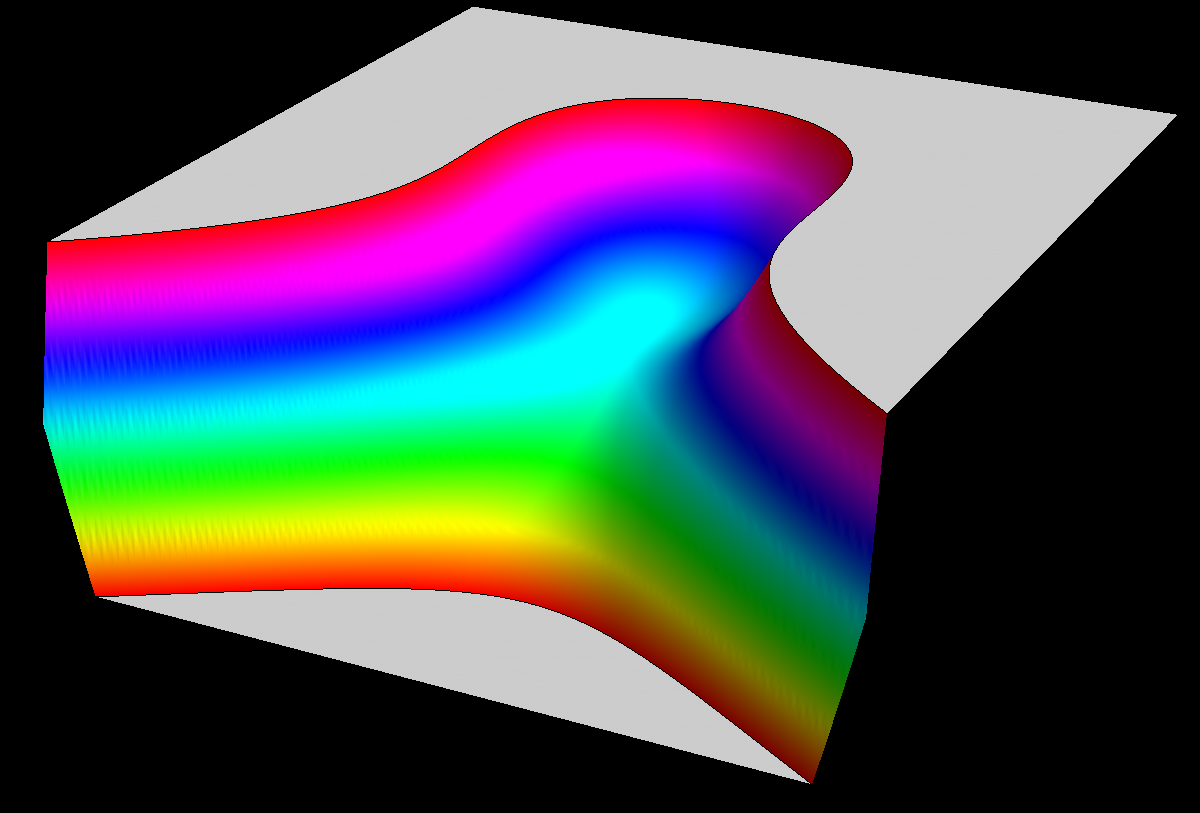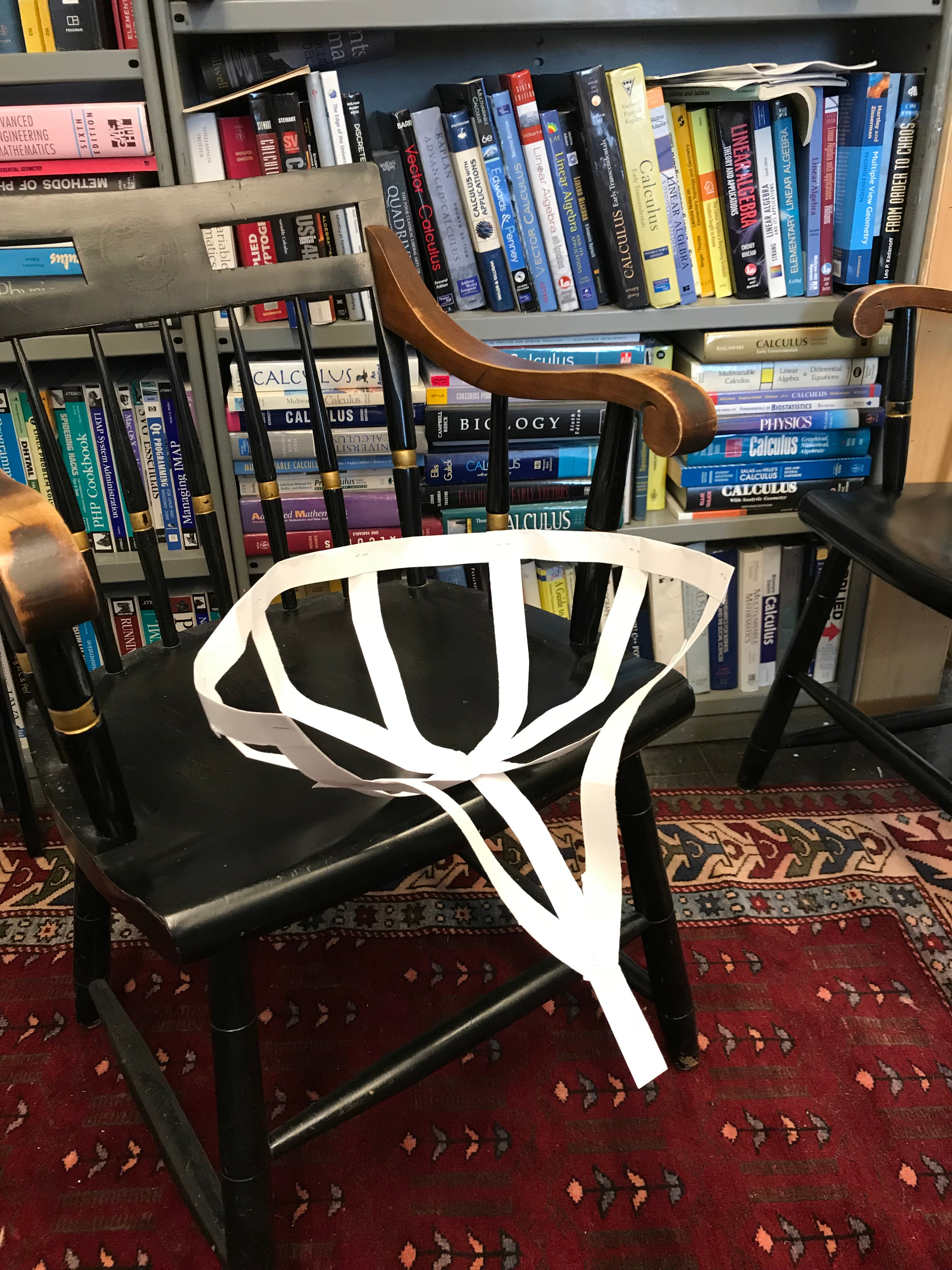## Koji's Chair

Question: this chair is the graph of some function f(x,y). Prize question: is D positive, negative or zero? And here is a bonus question due to Jun Hou: if a function f(x,y) is given for which in one direction we see the x^3 graph. What values are possible for D? Can it be positive, negative or zero?Here is the chair built by Koji